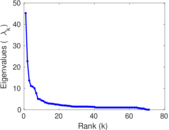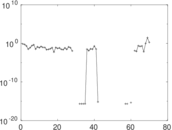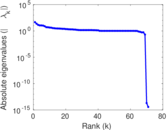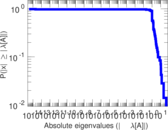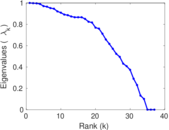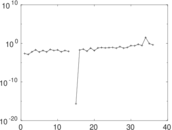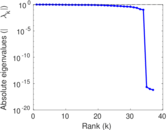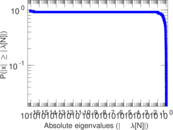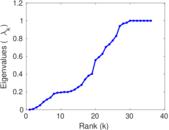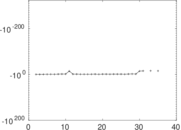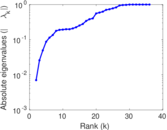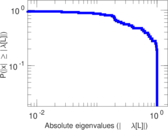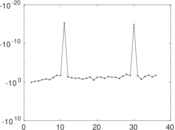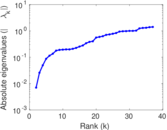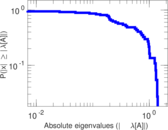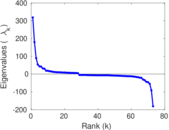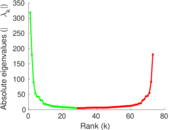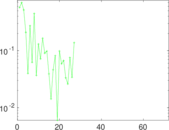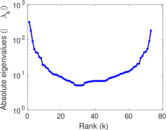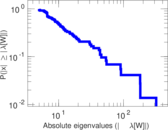# Wikibooks edits (co)

This is the bipartite edit network of the Corsican Wikibooks. It contains users and pages from the Corsican Wikibooks, connected by edit events. Each edge represents an edit. The dataset includes the timestamp of each edit.

 Code `bco` Internal name `edit-cowikibooks` Name Wikibooks edits (co) Data source http://dumps.wikimedia.org/ AvailabilityDataset is available for download Consistency checkDataset passed all tests Category Authorship network Dataset timestamp 2017-10-20 Node meaning User, article Edge meaning Edit Network formatBipartite, undirected Edge typeUnweighted, multiple edges Temporal dataEdges are annotated with timestamps

## Statistics

 Size n = 329 Left size n1 = 73 Right size n2 = 256 Volume m = 500 Unique edge count m̿ = 328 Wedge count s = 5,186 Claw count z = 126,955 Cross count x = 2,698,212 Square count q = 273 4-Tour count T4 = 23,708 Maximum degree dmax = 94 Maximum left degree d1max = 94 Maximum right degree d2max = 45 Average degree d = 3.039 51 Average left degree d1 = 6.849 32 Average right degree d2 = 1.953 12 Fill p = 0.017 551 4 Average edge multiplicity m̃ = 1.524 39 Size of LCC N = 228 Diameter δ = 17 50-Percentile effective diameter δ0.5 = 6.260 28 90-Percentile effective diameter δ0.9 = 11.751 9 Median distance δM = 7 Mean distance δm = 6.925 31 Gini coefficient G = 0.632 578 Balanced inequality ratio P = 0.256 000 Left balanced inequality ratio P1 = 0.212 000 Right balanced inequality ratio P2 = 0.332 000 Relative edge distribution entropy Her = 0.872 214 Power law exponent γ = 4.173 46 Tail power law exponent γt = 2.431 00 Tail power law exponent with p γ3 = 2.431 00 p-value p = 0.034 000 0 Left tail power law exponent with p γ3,1 = 2.051 00 Left p-value p1 = 0.496 000 Right tail power law exponent with p γ3,2 = 4.431 00 Right p-value p2 = 0.452 000 Degree assortativity ρ = −0.310 903 Degree assortativity p-value pρ = 8.799 71 × 10−9 Spectral norm α = 45.221 7 Algebraic connectivity a = 0.007 034 45 Spectral separation |λ1[A] / λ2[A]| = 1.990 32 Controllability C = 186 Relative controllability Cr = 0.574 074

## Plots

### Fruchterman–Reingold graph drawing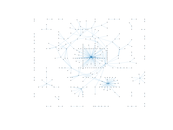### Degree distribution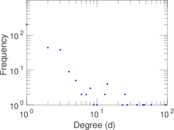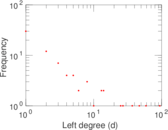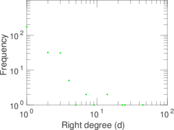### Cumulative degree distribution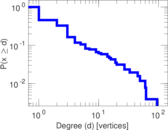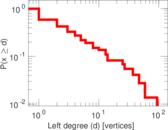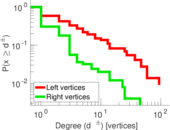### Lorenz curve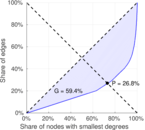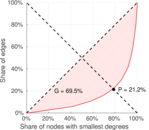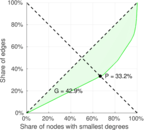### Spectral distribution of the adjacency matrix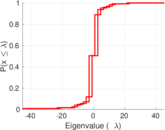### Spectral distribution of the normalized adjacency matrix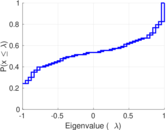### Spectral distribution of the Laplacian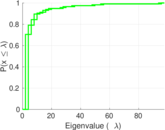### Spectral graph drawing based on the adjacency matrix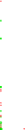### Spectral graph drawing based on the Laplacian### Spectral graph drawing based on the normalized adjacency matrix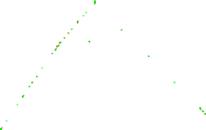### Degree assortativity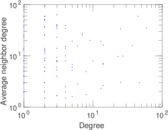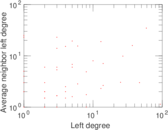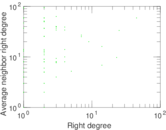### Zipf plot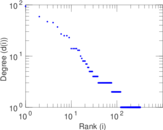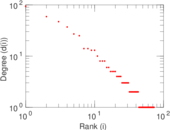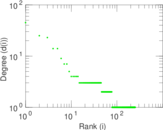### Hop distribution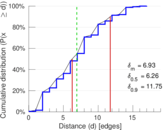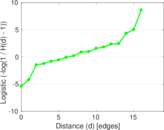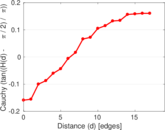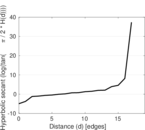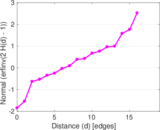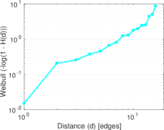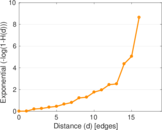### Double Laplacian graph drawing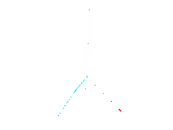### Delaunay graph drawing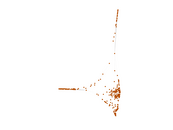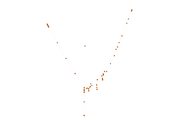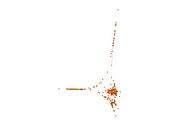### Edge weight/multiplicity distribution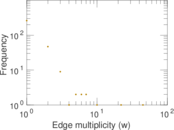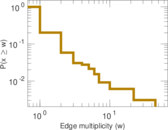### Temporal distribution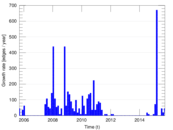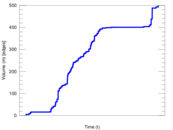### Temporal hop distribution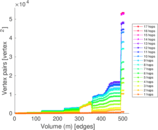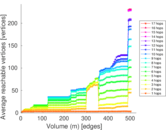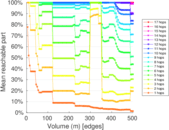### Diameter/density evolution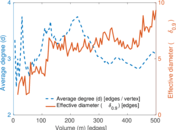### Matrix decompositions plots